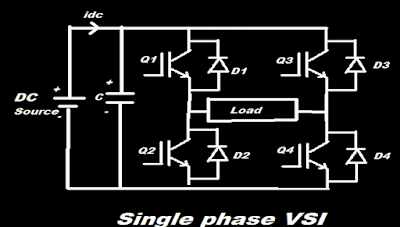# What is Voltage Source Inverter (VSI) ?

173 views
What is Voltage Source Inverter (VSI) ?

Voltage Source Inverter (VSI)•Inverter is device which converts Direct Current (DC) to Alternating Current (AC).

•There are two types of inverters one is Voltage source inverter (VSI) and second is current source inverter (CSI).

•Voltage source inverter keeps the voltage constant.

•Current source inverter keeps the current constant.

•Voltage source inverter (VSI) consists of one dc voltage source, transistors for switching, and one  large dc link capacitor.

•DC source may be a battery, Dynamos or Solar cell.

•Transistor may be IGBT, BJT, MOSFET, or GTO.

•For Direct Current the large DC link capacitor acts like open circuit and for high frequency AC the capacitive impedance is low.

•VSI may be single phase bridge circuit or three phase bridge circuit.

•Single phase bridge circuit consist of two legs of switches each leg consist of upper and lower switch.

•Antiparallel diode is use to allow non-unity power factor load at output.

•The single phase VSI consist of two load terminals and three phase VSI consist of three load terminals.

## Related Questions

359 views

Description : Give the circuit analysis of current source inverter with resistive load. What are the main difference between voltage source inverter and current source inverter?

Last Answer : Current source inverter (CSI): In current source inverter, the output current is independent of load. The input current of current source inverter is constant. Current source converter converts DC input current to ... are connected in parallel. In CSI diodes are connected in series.

3,479 views

Description : A voltage source inverter will have better performance if its  (1) load inductance is small and source inductance is large.  (2) both load and source inductance are small.  (3) both load and source inductances are large.  (4) load inductance is large and source inductance is small.

Last Answer : A voltage source inverter will have better performance if its load inductance is large and source inductance is small.

1,726 views

Description : A voltage source inverter is normally employed when  (1) source inductance is large and load inductance is small (2) source inductance is small and load inductance is large (3) both source and load inductance are small (4) both source and load inductance are large

156 views

Description : A 3-phase voltage source inverter operates in 180° conduction mode with a star connected resistive load. If input dc voltage is 100 V, then the peak to peak output line voltage is :

Last Answer : A 3-phase voltage source inverter operates in 180° conduction mode with a star connected resistive load. If input dc voltage is 100 V, then the peak to peak output line voltage is : 200 V

474 views

Description : Explain the three phase 120 degree mode VSI.

Last Answer : Three phase 120 degree mode VSI: In the 120-degree mode voltage source inverter, each thyristor conducts for 120 degree of a cycle. 120-degree mode VSI required six steps, each step of 60 degree ... conduct from the upper group and one thyristor will conduct from lower group.

789 views

Description : Justify. Power MOSFET is operated at high enough gate source voltage to minimize the conduction losses.

Last Answer : MOSFET is metal oxide field effect transistor. MOSFET is a voltage controlled device. MOSFET is majority carrier device. MOSFET are of two one is n-channel MOSFET and p-channel MOSFET. Gate, ... . Hence, power MOSFET is operated at high enough gate-source voltage to minimize the conduction losses.

157 views

Description : Nodal Analysis with Voltage Sources dependent voltage source and two supernode

Last Answer : Nodal Analysis with Voltage Sources dependent voltage source and two supernode

132 views

Description : Nodal analysis example with voltage source and supernode

Last Answer : Nodal analysis example with voltage source and supernode

56 views

Description : Find power delivered by voltage source

Last Answer : Find power delivered by voltage source